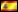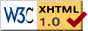Invited signatures

# Symbolic computation with C# 3.0

#####Versión en español

This practical article shows the possibilities for symbolic manipulation that open before us thanks to the availability of expression trees in C# 3.0.

### 1. Introduction

In our previous installment  we introduced C# 3.0 expression trees and showed how they can be used to reflect the structure of expressions (functions) over one or more parameters (variables). Thinking a little bit more about them, one can realize that the availability of this class hierarchy in .NET Framework open new doors for an efficient and more or less comfortable implementation of the symbolic manipulation of expressions, a task that previously was generally reserved to languages like LISP, where the uniform representation of code and data makes a lot easier their transformation in both directions.

In this article, we present a possible implementation of a library for the calculation of derivatives of mathematical functions, a classic example of symbolic computation. For a basic presentation of the concept of derivative, we direct the reader to ; here will be enough to say that the derivative of a function f(x) (for instance, x + sin(x)) is another function (in this case, 1 + cos(x)) that is obtained by means of applying a set of symbolic manipulation rules like, for instance, the sum rule: the derivative of a sum equals the sum of the derivatives of the operands.

### 2. Implementation of the library

The task we must solve here is the implementation of a library that allows us, given an expression tree (an instance of the class Expression<T>) to obtain another object of the same class that represents the derivative of the original expression. Let’s call Derive() the  (overloaded) method that will calculate the derivative; for its implementation, we will leverage another new feature in C# 3.0: extension methods . Thanks to the use of extension methods, we will be able to invoke Derive() over any expression using the natural syntax of object orientation:

```// expr is of type Expression<Func<double, double>>
Console.WriteLine(expr.Derive());```

```// expr is of type Expression<Func<double, double>>
// ExpressionExtensions is the class that contains Derive()
Console.WriteLine(ExpressionExtensions.Derive(expr));```

The source code of the library has the following structure:

```namespace Pokrsoft.Expressions
{
public static class ExpressionExtensions
{
public static Expression<T> Derive<T>(this Expression<T> e)
{
// …
}

public static Expression<T> Derive<T>(this Expression<T> e,
string paramName)
{
// …
}
private static Expression Derive(this Expression e, string paramName)
{
// …
}

}

public class ExpressionExtensionsException: Exception
{
public ExpressionExtensionsException(string msg) : base(msg, null) { }
public ExpressionExtensionsException(string msg, Exception innerException) :
base(msg, innerException) { }
}
}
```

The ExpressionExtensions class offers two variants of Derive():

• One with an only argument, the expression to derive. This variant assumes that the expression has exactly one variable. Note the presence of the this modifier before the type of the method parameter; that modifier identifies the method as an extension method for that type.
• Another variant with a second parameter, the name of the variable to use for taking the derivative. This variant allows us to implement the so called partial derivatives; basically, if a function has several variables, one could calculate the derivative considering any one these variables of them as “the variable” and the other ones as constant values.

The first variant is implemented like this:

```public static Expression<T> Derive<T>(this Expression<T> e)
{
// check not null expression
if (e == null)
throw new ExpressionExtensionsException("Expression must be non-null");
// check just one param (variable)
if (e.Parameters.Count != 1)
throw new ExpressionExtensionsException("Incorrect number of parameters");
// check right node type (maybe not necessary)
if (e.NodeType != ExpressionType.Lambda)
throw new ExpressionExtensionsException("Functionality not supported");
// calc derivative
return Expression.Lambda<T>(e.Body.Derive(e.Parameters.Name),
e.Parameters);
}
```

After rejecting the incorrect cases, the method calls another extension method also called Derive(), but this time for the non-generic Expression class we presented in , passing to it the body of the expression, available through the Body property of the original instance.

The extension method for the Expression class (which we have declared as private in order to make it invisible to outside code) is the real “power horse” that implements, in a recursive manner, all the functionality related to the computation of derivatives. Inside this method you can find coded the different rules described in  for the different possible types of expression nodes. A fragment of the implementation follows:

```private static Expression Derive(this Expression e, string paramName)
{
switch (e.NodeType)
{
// constant rule
case ExpressionType.Constant:
return Expression.Constant(0.0);
// parameter
case ExpressionType.Parameter:
if (((ParameterExpression) e).Name == paramName)
return Expression.Constant(1.0);
else
return Expression.Constant(0.0);
// sign change
case ExpressionType.Negate:
Expression op = ((UnaryExpression) e).Operand;
return Expression.Negate(op.Derive(paramName));
// sum rule
{
Expression dleft =
((BinaryExpression) e).Left.Derive(paramName);
Expression dright =
((BinaryExpression) e).Right.Derive(paramName);
}
// product rule
case ExpressionType.Multiply:
{
Expression left = ((BinaryExpression) e).Left;
Expression right = ((BinaryExpression) e).Right;
Expression dleft = left.Derive(paramName);
Expression dright = right.Derive(paramName);
Expression.Multiply(left, dright),
Expression.Multiply(dleft, right));
}
// *** other node types here ***
default:
throw new ExpressionExtensionsException(
"Not implemented expression type: " + e.NodeType.ToString());
}
}
```

After adding a reference to this library in any type of LINQ-related project, we’ll only need to import the Pokrsoft.Expressions namespace, and then will be able to take the derivative of functions in the following way:

```Expression<Func<double, double>> circleAreaExpr =
Console.WriteLine(circleAreaExpr.Derive());
```

The output generated by this code (indented for a better understanding) will be:

```    radius => Add(
Multiply(
Multiply(
3,14159265358979,
1),
Multiply(
Multiply(
3,14159265358979,
1),
Multiply(
0,
```

that after the convenient simplifications (see next section) will become 2 * Math.PI * radius, the correct result.

### 3. Further work

A full implementation of a method for the calculation of derivatives is not a simple task, and the version we offer with this article is not complete; mainly, there remains some work to do regarding the programming of the derivatives of elementary functions (logarithmic, trigonometric, etc.) that can appear in an expression. In this regard and as an example, we have provided an implementation of the well known power rule :

```case ExpressionType.MethodCall:
Expression e1 = null;
MethodCallExpression me = (MethodCallExpression) e;
MethodInfo mi = me.Method;

// TEMPORARY
// method should be static and its class - Math
if (!mi.IsStatic || mi.DeclaringType.FullName != "System.Math")
throw new ExpressionExtensionsException("Not implemented function: " +
mi.DeclaringType + "/" + mi.Name);

switch (mi.Name)
{
case "Pow":
// power rule
e1 =
Expression.Multiply(
parms,
Expression.Call(mi, null,
new Expression[] {
parms,
Expression.Subtract(
parms,
Expression.Constant(1.0)) }));
break;
default:
throw new ExpressionExtensionsException("Not implemented function: " +
mi.Name);
}
// chain rule
return Expression.Multiply(e1, parms.Derive(paramName));
```

A small inconvenience in the current implementation of expression trees is the absence of a node type for the operation “raise to power”; for this reason, we’ve had to assume that this operation will be expressed by means of a call to the method Math.Pow().

Another pending task we can recommend to the reader as a good exercise is the implementation of an extension method for the simplification y factorization of expressions. The programming of such simple rules as 0 + y = y or 1 * y = y for any given y would have allowed us to show a much readable expression in the previous section.

Furthermore, a task a bit more complex, but maybe much more interesting, is that of graphically rendering an expression tree, using the traditional symbols of mathematical notation.

### 4. Conclusions

In this very practical article we have shown one of the many possibilities that open before us thanks to the availability of expression trees in C# 3.0.

The source code of the example can be downloaded from this site. In order to run it, the May 2006 LINQ Preview, available at , must be downloaded and installed.

### 5. References

1. C# 3.0 and LINQ related resources: http://msdn.microsoft.com/csharp/future/default.aspx.
2. Hernández, Octavio “What Orcas will bring: new features in C# 3.0”, published in dotNetManía Nº 24, March 2006 (in Spanish).
3. 3. Hernández, Octavio “Expression trees in C# 3.0”, in  http://www.elguille.info/NET/futuro/firmas_octavio_ArbolesExpresiones_EN.htm, January 2007.
4. “Derivative”, in Wolfram MathWorld, http://mathworld.wolfram.com/Derivative.html

 Sample code (ZIP): File with sample code: Octavio_Derivadas.zip - 3.79 KB (MD5 checksum: 048B3E07938B3D73DA9944B57729B48E)